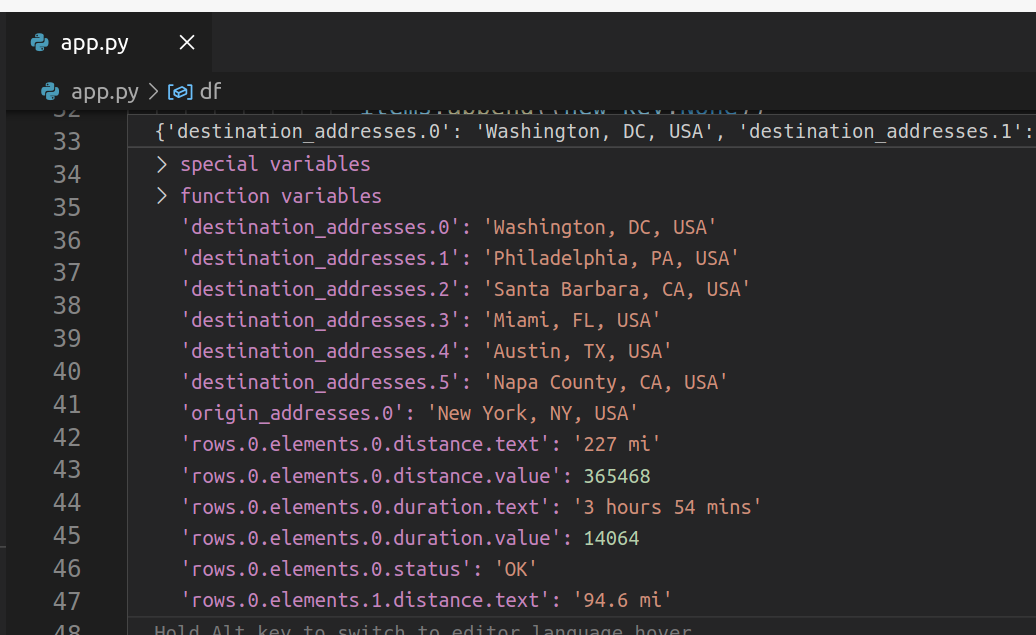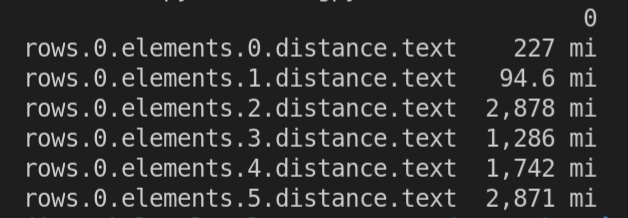# Handling deeply nested JSON with Python

Lee Hawthorn June 01, 2021 #Python

I first came across JSON back in 2015. As I predicted back then it's taken off in a huge way due to the growth in node based back ends and growth in JavaScript.

I do miss the structure of SQL databases.

To process JSON with Python we can use a list or dict comprehension, however, custom code is typically needed for different JSON data.

Another option is to flatten JSON to a key value dictionary.

I use a flatten function to do this. Thanks to a stack overflow post.

I've been able to use the function below to process very deeply nested JSON.

Look at the JSON below - taken from the Google Maps Distance API.

``````{
"Washington, DC, USA",
"Santa Barbara, CA, USA",
"Miami, FL, USA",
"Austin, TX, USA",
"Napa County, CA, USA"
],
"rows": [
{
"elements": [
{
"distance": {
"text": "227 mi",
"value": 365468
},
"duration": {
"text": "3 hours 54 mins",
"value": 14064
},
"status": "OK"
},
{
"distance": {
"text": "94.6 mi",
"value": 152193
},
"duration": {
"text": "1 hour 44 mins",
"value": 6227
},
"status": "OK"
},
{
"distance": {
"text": "2,878 mi",
"value": 4632197
},
"duration": {
"text": "1 day 18 hours",
"value": 151772
},
"status": "OK"
},
{
"distance": {
"text": "1,286 mi",
"value": 2069031
},
"duration": {
"text": "18 hours 43 mins",
"value": 67405
},
"status": "OK"
},
{
"distance": {
"text": "1,742 mi",
"value": 2802972
},
"duration": {
"text": "1 day 2 hours",
"value": 93070
},
"status": "OK"
},
{
"distance": {
"text": "2,871 mi",
"value": 4620514
},
"duration": {
"text": "1 day 18 hours",
"value": 152913
},
"status": "OK"
}
]
}
],
"status": "OK"
}
``````

Here's the recursive function I use for different json sources.

``````def flatten(dictionary, parent_key=False, separator='.', log=False):
"""
Turn a nested dictionary into a flattened dictionary
:param dictionary: The dictionary to flatten
:param parent_key: The string to prepend to dictionary's keys
:param separator: The string used to separate flattened keys
:param log : Bool used to control logging to the terminal
:return: A flattened dictionary
"""

items = []
for key, value in dictionary.items():
if log: print('checking:',key)
new_key = str(parent_key) + separator + key if parent_key else key
if isinstance(value, collections.abc.MutableMapping):
if log: print(new_key,': dict found')
if not value.items():
items.append((new_key,None))
else:
items.extend(flatten(value, new_key, separator).items())
elif isinstance(value, list):
if log: print(new_key,': list found')
if len(value):
for k, v in enumerate(value):
items.extend(flatten({str(k): v}, new_key).items())
else:
items.append((new_key,None))
else:
items.append((new_key, value))
return dict(items)

``````

Calling this function transforms the json into a flat dict:Having done that it's simple to make any further transforms you need using a `pandas` dataframe.

Here's some sample code as an example to extract distance values. Python version greater than 3.3 is required to run the code below.

``````with open('data.json') as f:

# Flatten json to dict
a = flatten(data)

df = pd.DataFrame.from_dict(a, orient='index', dtype=str)

# Filter accordingly
df = df[df.index.str.contains("distance.text")]
pprint(df)
``````Here's the complete code:

``````import json
import pandas as pd
from pprint import pprint
import collections.abc

def flatten(dictionary, parent_key=False, separator='.', log=False):
"""
Turn a nested dictionary into a flattened dictionary
:param dictionary: The dictionary to flatten
:param parent_key: The string to prepend to dictionary's keys
:param separator: The string used to separate flattened keys
:param log : Bool used to control logging to the terminal
:return: A flattened dictionary
"""

items = []
for key, value in dictionary.items():
if log: print('checking:',key)
new_key = str(parent_key) + separator + key if parent_key else key
if isinstance(value, collections.abc.MutableMapping):
if log: print(new_key,': dict found')
if not value.items():
items.append((new_key,None))
else:
items.extend(flatten(value, new_key, separator).items())
elif isinstance(value, list):
if log: print(new_key,': list found')
if len(value):
for k, v in enumerate(value):
items.extend(flatten({str(k): v}, new_key).items())
else:
items.append((new_key,None))
else:
items.append((new_key, value))
return dict(items)

with open('data.json') as f: